## RS Aggarwal Class 6 Solutions Chapter 2 Factors and Multiples Ex 2C

These Solutions are part of RS Aggarwal Solutions Class 6. Here we have given RS Aggarwal Solutions Class 6 Chapter 2 Factors and Multiples Ex 2C.

Other Exercises

Give the prime factorization of each of the following numbers.

Question 1.
Solution:
We have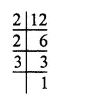∴12 = 2 x 2 x 3 = 22 x 3.

Question 2.
Solution:
We have∴18 = 2 x 3 x 3 = 2 x 32

Question 3.
Solution:
We have∴ 48 = 2 x 2 x 2 x 2 x 3
= 24 x 3.

Question 4.
Solution:
We have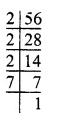∴ 56 = 2 x 2 x 2 x 7
= 23 x 7.

Question 5.
Solution:
We have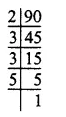∴ 90 = 2 x 3 x 3 x 5
= 2 x 32 x 5.

Question 6.
Solution:
We have136 = 2 x 2 x 2 x 17
= 23 x 17.

Question 7.
Solution:
We have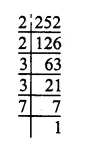∴ 252 = 2 x 2 x 3 x 3 x 7
= 22 x 32 x 7.

Question 8.
Solution:
We have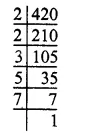∴ 420 = 2 x 2 x 3 x 5 x 7
= 22 x 3 x 5 x 7.

Question 9.
Solution:
We have∴ 637 = 7 x 7 x 13
= 72 x 13.

Question 10.
Solution:
We have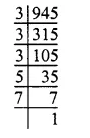∴ 945 = 3 x 3 x 3 x 5 x 7
= 33 x 5 x 7.

Question 11.
Solution:
We have∴ 1224 = 2 x 2 x 2 x 3 x 3 x 17
= 23 x 32 x 17.

Question 12.
Solution:
We have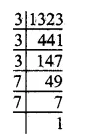∴ 1323 = 3 x 3 x 3 x 7 x 7
= 33 x 72

Question 13.
Solution:
We have∴ 8712 = 2 x 2 x 2 x 3 x 3 x 11 x 11
= 23 x 32 x 112.

Question 14.
Solution:
We have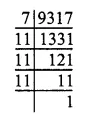∴9317 = 7 x 11 x 11 x 11
= 7 x 113

Question 15.
Solution:
We have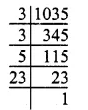∴ 1035 = 3 x 3 x 5 x 23
= 32 x 5 x 23

Question 16.
Solution:
We have∴ 1197 = 3 x 3 x 7 x 19
= 32 x 7 x 19

Question 17.
Solution:
We have∴ 4641 = 3 x 7 x 13 x 17.

Question 18.
Solution:
We have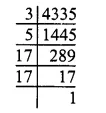∴ 4335 = 3 x 5 x 17 x 17
= 3 x 5 x 172

Question 19.
Solution:
We have∴ 2907 = 3 x 3 x 17 x 19
= 32 x 17 x 19.

Question 20.
Solution:
We have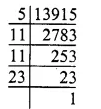∴ 13915 = 5 x 11 x 11 x 23 = 5 x 112 x 23

Hope given RS Aggarwal Solutions Class 6 Chapter 2 Factors and Multiples Ex 2C are helpful to complete your math homework.

If you have any doubts, please comment below. Learn Insta try to provide online math tutoring for you.